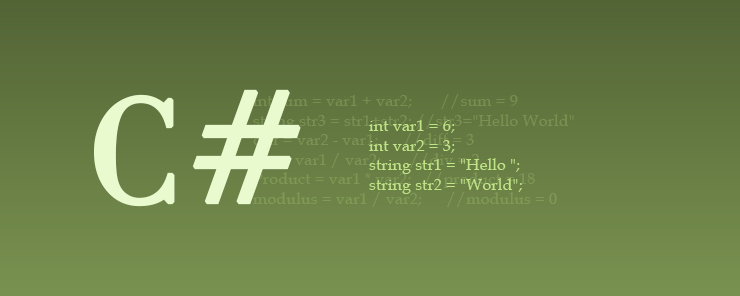# Learn about Expressions in C# Programming

0
3046As in other programming languages, C# programs consist of statements. Statements can be defined as the building blocks of computer programs. The statement itself consists of one or more expressions. In this article, we are going to tackle this important topic. So bear with us, and you will really like it.

Expressions
An expression is the result of combining one or more variable with an operator. C# offers the following kinds of operators: Arithmetic, Assignment, and Logical.

Arithmetic Operators
They work not only with numeric values (as you might guess), but they could also work with strings as well. Moreover, they could be used in variable assignment. Let’s assume we have the following variables:

```int var1 = 6;
int var2 = 3;
string str1 = "Hello ";
string str2 = "World";
```

Consider the following table:

 Operator Uses Example + Adds two numeric variables. Concatenates two or more strings. int sum = var1 + var2;       //sum = 9 string str3 = str1+str2; //str3=”Hello World” – Subtracts the right side operand from the left side one. diff = var2 – var1;      //diff = 3 / Divides two numeric variables div = var1 / var2;       //div = 2 * Multiplies two numeric variables Product = var1 * var2;   //product = 18 % Returns the remainder of dividing two numeric variables modulus = var1 / var2;      //modulus = 0

Besides to the “ordinary” arithmetic operators shown in the above table, C# also offers a special type of arithmetic operators: the increment and decrement operators:

 Operator Uses Example ++ The value of the variable is incremented by 1 var2=var1++; //var2 = 7, var1= 7 var2++;      //var2 = 8 — The value of the variable is decremented by 1 var2=var1–; //var2 = 5, var1=5

Operator Precedence
Let’s add one more variable to our example:

`int var3 = 5;`

Now consider the following statements:

```int output;
output = var1 + var2 * var3;
Console.WritleLine(output);
```

What do you think output will be?

If you thought it would be 45, then I am sorry to disappoint you!! C# uses operator precedence to calculate arithmetic operations. This means that the multiplication is evaluated first (var2 * var3 = 3, 3 * 5 = 15), then the product is added to var1. So it would be 6 + 15 = 21, and this would be the value of output.

So, take it as a rule:

Whenever you perform arithmetic operations in C#, bear in mind that multiplication, division, and modulus are evaluated before addition and subtraction.

However, you can always control what gets evaluated first in your equation by using parentheses. So if you want the previous example to evaluate the addition, then the multiplication (that will produce 45), you need to re-write it as follows:

```int output;
output = (var1 + var2) * var3;
Console.WritleLine(output);
```

So, another important point to remember: expressions between parentheses have higher precedence (and get evaluated first) than other expressions.Assignment operators
If you thought the only assignment operator used in C# is the ordinary equal sign ‘=’, then it might surprise you to know that the language contains five more assignment operators that offer you even more power and flexibility when coding. Have a look at the following table:

 Operator Uses Example = Assigns the value (or the result of expression) on the right side to the variable on the left side. var1=var2; //both contain the same value now += Adds the right side operand to the left side one, and then assigns the result to the left side operand. var1+= var2; //the same as var1 = var1 + var2 -= Subtracts the right side operand from the left side one. The result of subtraction is then assigned to the left operand. var1 -= var2; //the same as var1 = var1 – var2 *= Multiplies the two operands, and assigns the result to the left side operand. var1 *= var2; //the same as var1 = var1 * var2 /= Divides the left side operand by the right side one. The result is then assigned to the left side operand. var1 /= var2; //the same as var1 = var1 / var2 %= Divides the left side operand by the right side one. The remainder of the operation is then assigned to the left side operand. var1 %= var2; //the same as var1 = var1 % var2

Summary

• Statements are the building blocks of computer programs.
• A statement consists of one or more expressions.
• Expressions could be assignment, arithmetic, or logical.
• Variables and operators form expressions.
• Arithmetic operators perform the basic arithmetic calculations.
• C# has a set of Assignment operators like = , += , -= , *= , /= , and %=
• C# borrowed the increment and decrement operators (++ and –) from the C/C++ languages.

In the next article, we will continue with operators, by discussing the Comparison and the Logical operators, and their usage in Decision Making. This will be an introduction before welcoming the superstar of the next article: the if statement. A rich article it is going to be, so don’t miss it!!!